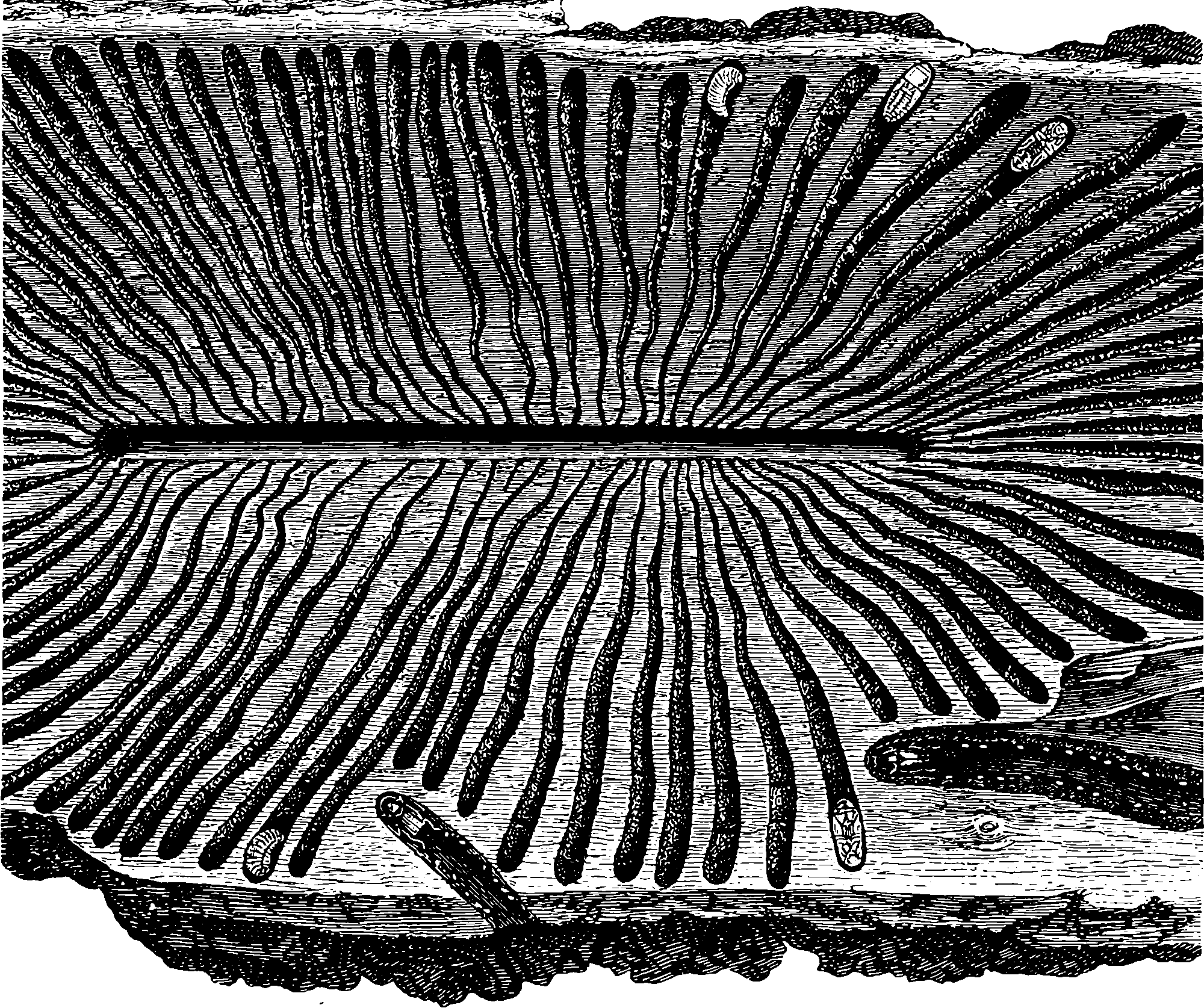# Neural nets with basis decomposition layersNeural networks incorporating basis decompositions.

Why might you want to do this? For one it is a different lense through which to analyze neural nets’ mysterious success through. For another, it gives you interpolation for free. Also this idea is part of the connection between neural nets and low rank GPs. There are possibly other reasons - perhaps the right basis gives you better priors for understanding a partial differential equation? Or something else?

## Unrolling: Implementing sparse coding using neural nets

Often credited to Gregor and LeCun (2010), this trick imagines each step in an iterative sparse coding optimisation as a layer in a neural net and then optimises the gradient descent step of that iterative coding, given you, in effect, a way of learning optimally fast, or optimally fast, sparse bases. This has been taken a long way by, e.g. Monga, Li, and Eldar (2021).

## Convolutional neural networks as sparse coding

Elad and Papyan and others have a miniature school of Deep Learning analysis based on Multi Layer Convolutional Sparse Coding . The argument here is that essentially Convnets are already solving sparse coding problems they just don’t know it. They argue:

The recently proposed multilayer convolutional sparse coding (ML-CSC) model, consisting of a cascade of convolutional sparse layers, provides a new interpretation of convolutional neural networks (CNNs). Under this framework, the forward pass in a CNN is equivalent to a pursuit algorithm aiming to estimate the nested sparse representation vectors from a given input signal. …Our work represents a bridge between matrix factorization, sparse dictionary learning, and sparse autoencoders, and we analyze these connections in detail.

However, as interesting as this sounds, I am not deeply engaged with it, since this does not solve any immediate problems for me.

## Continuous basis functions

Convnet require a complete rasterized grid, but often signels are not observed on a regular grid. This is precisely the problem of signal sampling. With basis functions of continuous support and a few assumptions it is tempting to imagine we can get neural networks which operate in a continuous space.. Can I uses continuous bases in the computation of a neural net? If so, this could be useful in things like learning PDEs. The virtue of these things is that they do not depend (much?) upon the scale of some grid. Possibly this naturally leads to us being able to sample the problem very sparsely. It also might allow us to interpolate sparse solutions. In addition, analytic basis functions are easy to differentiate; we can use autodiff to find their local spatial gradients, even deep ones.

There are various ways other to do native interpolation; One hack uses the implicit representation method which is a clever trick — in that setting we reuse the autodiff architecture to calculate gradients with respect to the output index, but not plausible for every problem, where something better behaved like a basis function interpretation is more helpful.

Specifically, I would like to do Bayesian inference which looks extremely hard through an implicit net, but only very hard through a basis decomposition.

In practice, how would I do this?

Using a well-known basis, such as orthogonal polynomial or Fourier bases, creating a layer which encodes your net is easy. After all, that is just an inner product. That is what methods like that of Li et al. (2020) exploit.

More general, non-orthogonal bases such as sparse/overcomplete frames might need to solve a complicated sparse optimisation problem inside the network.

One approach is presumably to solve the basis problem in implicit layers.1 Differentiable Convex Optimization Layers introduces cvxpylayers; perhaps that does some of the work we want?

I would probably not attempt to learn an arbitrary sparse basis dictionary in this context, because that does not interpolate naturally, but I can imagine learning a parametric sparse dictionary, such as one defined by some simple basis such as decaying sinusoids.

How would wavelet decompositions fit in here?

1. Not to be confused with implicit representation layers which are completely different.↩︎

### No comments yet. Why not leave one?

GitHub-flavored Markdown & a sane subset of HTML is supported.